# Wire D

Wire length 1 m is bent so that it forms a semicircle circuit (including the diameter). Determine the radius of the semicircle.

Correct result:

r =  0.19 m

#### Solution:

$r = \dfrac{ 1}{2+\pi} = 0.19 \ \text{m}$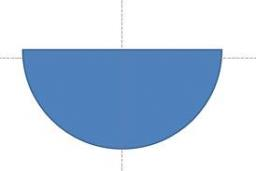We would be pleased if you find an error in the word problem, spelling mistakes, or inaccuracies and send it to us. Thank you!Tips to related online calculators
Do you want to convert length units?

#### You need to know the following knowledge to solve this word math problem:

We encourage you to watch this tutorial video on this math problem:

## Next similar math problems:

• Snowman 2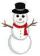On the medal, which has the shape of a circle with a diameter 18 cm is sketched snowman so that the following requirements are met: 1. snowman is composed of three circles, 2. space over snowman is the same as under it, 3. diameters of all circles express
• Snowman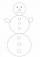In a circle with a diameter 50 cm are drawn 3 circles /as a snowman/ where: its diameters are integers, each larger circle diameter is 3 cm larger than the diameter of the previous circle. Determine snowman height if we wish highest snowman.
• RectangleThe rectangle is 21 cm long and 38 cm wide. Determine the radius of the circle circumscribing rectangle.
• Cube in a sphereThe cube is inscribed in a sphere with volume 7253 cm3. Determine the length of the edges of a cube.
• MovementFrom the crossing of two perpendicular roads started two cyclists (each at different road). One runs at average speed 28 km/h, the second at average speed 24 km/h. Determine the distance between them after 45 minutes cycling.
• Rope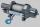How many meters of rope 10 mm thick will fit on the bobbin diameter of 200 mm and length 350 mm (central mandrel have a diameter 50 mm)?
• Rhombus and inscribed circleIt is given a rhombus with side a = 6 cm and the radius of the inscribed circle r = 2 cm. Calculate the length of its two diagonals.
• Concentric circlesIn the circle with diameter 19 cm is constructed chord 9 cm long. Calculate the radius of a concentric circle that touches this chord.
• SectorThe perimeter of a circular sector with an angle 1.8 rad is 64 cm. Determine the radius of the circle from which the sector comes.
• Velocipedes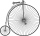In the 19th century, bicycles did not have a chain drive, and the pedals were connected directly to the wheel axis. This wheel diameter gradually increased until the so-called high bicycles (velocipedes) with a front wheel diameter of up to 1.5 meters, wh
• Concentric circles and chordIn a circle with a diameter d = 10 cm, a chord with a length of 6 cm is constructed. What radius have the concentric circle while touch this chord?
• ForcesIn point O acts three orthogonal forces: F1 = 20 N, F2 = 7 N and F3 = 19 N. Determine the resultant of F and the angles between F and forces F1, F2 and F3.
• Quarter circularThe wire that is hooked around the perimeter of quarter-circular arc has length 3π+12. Determine the radius of circle arc.
• The fenceI'm building a fence. Late is rounded up in semicircle. The tops of late in the field between the columns are to copy an imaginary circle. The tip of the first and last lath in the field is a circle whose radius is unknown. The length of the circle chord
• CirclesThe areas of the two circles are in the ratio 2:20. The larger circle has diameter 20. Calculate the radius of the smaller circle.
• ConeCircular cone of height 15 cm and volume 5699 cm3 is at one-third of the height (measured from the bottom) cut by a plane parallel to the base. Calculate the radius and circumference of the circular cut.
• Five circlesOn the line segment CD = 6 there are 5 circles with radius one at regular intervals. Find the lengths of the lines AD, AF, AG, BD, and CE